# Aggregation

Learn how Cognite Data Fusion aggregates and interpolates time series data, and see the details about the available aggregation functions.

## Aggregation in Cognite Data Fusion​

To improve performance and to reduce the amount of data transferred in query responses, Cognite Data Fusion pre-calculates the most common aggregates for numerical data points in time series. These aggregates are available with millisecond response time even when you are querying across large data sets.

In your queries, you can specify one or more aggregates (for example `average`, `minimum` and `maximum`) and also the time granularity for the aggregates (for example `1h` for one hour).

Aggregates are aligned to the start time modulo of the granularity unit. If you ask for daily average temperatures since Monday afternoon last week, the first aggregated data point will contain averages for the whole of Monday, the second for Tuesday, etc.

NOTE

Cognite Data Fusion determines aggregate alignment based only on the granularity unit. If you specify hour aggregates, and the start time of the request is in the middle of the hour, the start time will round up to the start time of the hour.

As a result, you can get different results if you aggregate over 60 minutes than if you aggregate over 1 hour because the two queries are aligned differently. For example, if the start time is `3:43:25`: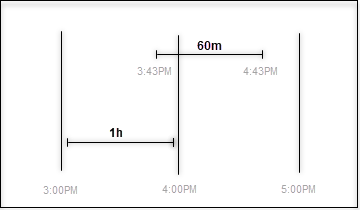### Aggregating data points​

Aggregation is to group together the values of many data points to form a single summary value. For example, the `count` aggregate gives the number of data points for a time range. The timestamp of the aggregate marks the beginning of the time range.

### Interpolating data points​

Interpolation is to construct new data points within the range of a discrete set of known data points. The returned data points have a timestamp and a value, where the value represents the interpolated value at the time of the timestamp. The interpolation method depends on whether the time series is stepwise or continuous. Interpolated data points aren't stored and are only visible as the aggregation/interpolation results.

### Stepwise vs continuous​

Interpolation and aggregation depend on how the time series is interpreted between the stored data points. A stepwise time series is assumed to keep its last reported value until a new value comes in, and then immediately jump to that new value. A continuous time series is assumed to gradually change between the stored data points and is modeled with linear interpolation.

Data points Stepwise interpretation Continuous interpretation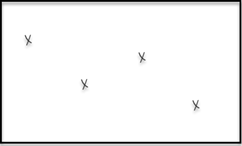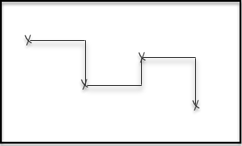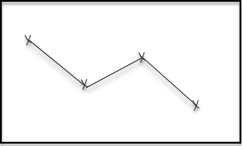How the times series is interpreted affects the value of aggregates. For example, the `average` aggregate, which is based on the average distance to zero, will be calculated as the area below the curve, divided by the size of the time range.

Stepwise interpretation Continuous interpretation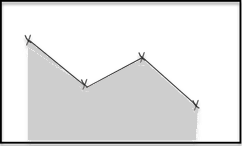### Granularity​

Granularity defines the time range that each aggregate is calculated from. It consists of a time unit and a size. Valid time units are `day` or `d`, `hour`or `h`, `minute` or `m` and `second` or `s`, for example, `2h` means that each time range should be 2 hours wide, `3m` means 3 minutes.

The value of an aggregate for a time range may also depend on data outside of the time range because lines have to be drawn to the edge of the time range to compute the aggregates.

Data points Stepwise interpretation Continuous interpretation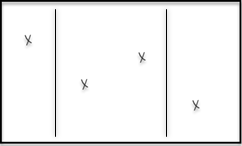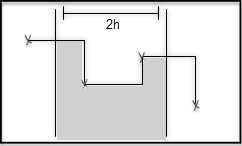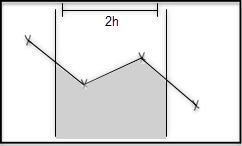### Missing data​

CDF doesn't return aggregates or interpolations for time ranges that have no data points, even if there are previous and next data points present for that period. As a result, the returned aggregates may skip large periods of time if the underlying data is sparse.

### Previous and next data point​

To interpolate a time series to the edges of the time range, many aggregates and interpolations depend on knowing the last data point before the time range, and the first data point after.

For continuous time series, CDF doesn't use the previous and next data points if they're more than one hour away from the time range. This is to avoid interpolating data when the underlying sensor has been down for an extended period of time.

For stepwise series, CDF uses the previous and next data points regardless of how distant they are.

We do not extrapolate backward from the first point in a time series or forward from the last point. This is also the case in stepwise time series, even though they are assumed to continuously maintain the value of the previous data point until the next point appears. The rationale behind this is that we can not know the reason that the sensor isn't sending new data: it could be because the value is unchanged, or because the sensor is down. We want to avoid implying that the sensor is always up.

## Aggregation functions​

To use the aggregation functions, you construct requests that look like this:

``    POST /api/v1/projects/{project}/timeseries/data/list    Host: api.cognitedata.com    api-key: <api-key>    Content-Type: application/json    {        "items": [            {            "limit": 10000,            "externalId": "your external id",            "aggregates": ["aggregate function 1","aggregate function 2"],            "granularity": "1h",            "start": 1541424400000,            "end":"now"            }        ]    }``

Cognite Data Fusion (CDF) supports the aggregation functions described below.

FunctionHow it’s calculatedWhen to use
averageIntegral of time series divided by the size of time rangeDownsampling many noisy RAW data points.
maxThe highest value of all stored data points.
minThe lowest value of all stored data points.
countThe count of stored data points.
sumThe sum of values of all stored data points.
interpolationThe interpolated value at the start of each time range.Interpolating sparse irregular data to regularly spaced time series.
stepInterpolationThe interpolated value at the start of each time range, treating time series as stepwise.
continuousVarianceThe variance of the underlying function when assuming linear or step behavior between data points.Uneven spacing between data points, if interpolation is a good assumption.
discreteVarianceThe variance of the discrete set of data points, no weighting for density of points in time.Evenly spaced data points.
totalVariationThe sum of absolute differences between neighboring data points in a period.Data quality checks or outlier detection.

### average​

Data points Stepwise interpretation Continuous interpretationThe `average` function computes the time-weighted average value of the time series per time range (can differ significantly from `sum` and `count`). The value is defined as the integral of the time series divided by the length of the time range.

When calculating the average, there is a difference between the stepwise and continuous data when looking at the first or last data point. The stepwise data always extends to a beginning to a period, which means it's possibly reach prior the first or past the last data point. Continuous data starts at the first and ends at the last data point.

For a continuous time series, if there is no data previous/next to the time range, or that data is more than 1 hour away, CDF doesn't interpolate backwards/forwards, and the integral is only done on parts of the time range.

Continuous time series with no previous data points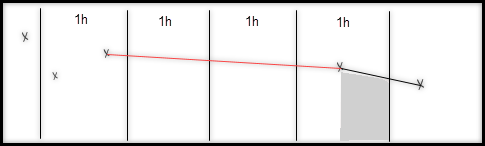### max​

For each time range, the `max` function returns the highest value of the stored data points in the time range.

max function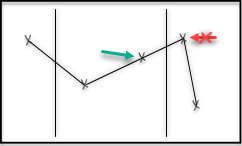The function doesn't include interpolated values at the edges of the time range. This means that the average can be greater than max.

### min​

For each time range, the `min` function returns the lowest value of all stored data points.

min function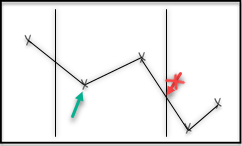The function doesn't include interpolated values at the edges of the time range.

### count​

The `count` function returns the number of data points for each time range. If there are no data points in a time range, this function returns no data.

count function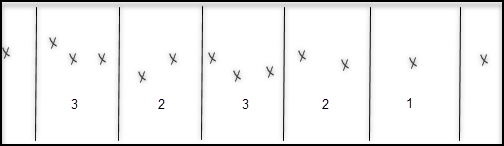### sum​

The `sum` function returns the sum of the values of all data points in the time range, or nothing if there are no data points.

sum function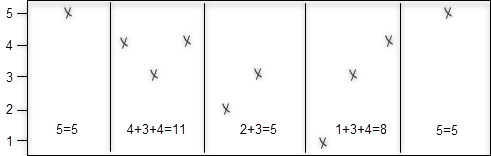### interpolation​

The `interpolation` function interpolates the value of the time series at the start of each time range. The method of interpolation is based on whether the time series is continuous or stepwise.

Note: For stepwise time series this is the same as the `stepInterpolation` function.

Data points Stepwise interpretation Continuous interpretation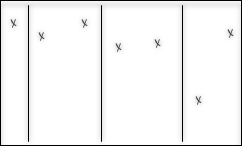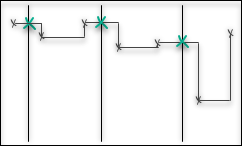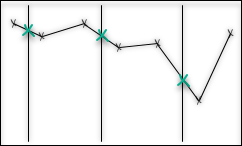### stepInterpolation​

Same as `interpolation`, but always treats the time series as stepwise.

### continuousVariance​

The variance of a function f is the expectation value of f squared, minus the square of the expectation value of f.

If CDF only has the value of f in a finite number of points, there are different approaches to approximate the variance. The continuous variance aggregate is intended for situations where the piecewise linear function that interpolates between the data points is a good approximation. If this function is f, CDF defines the continuous variance in a time period from t=a to t=b as: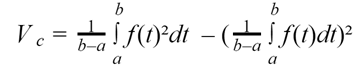The time intervals between data points can vary due to a sampling setting that tries to capture the behavior of f with a piecewise linear function (or a step function) using relatively few data points. These are cases when the continuous variance is a meaningful variance for the function. On the other hand, if the data points are sampled at even time intervals, independently of the value of f, the piecewise linear function will cut away extremal points, and CDF will get a variance lower than the actual variance.

### discreteVariance​

The `discreteVariance`function is for cases where the data points are measured at regular time intervals, independently of the values they measure. In these cases, CDF can regard the data points as a random sampling of the values in the time period. CDF defines the variance as: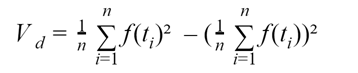### totalVariation​

The `totalVariation` function returns the total absolute change in the function values within a time interval. If the time interval goes from t=a to t=b with n data points, the total variation is defined as:CDF uses the interpolated values for f at a and b.